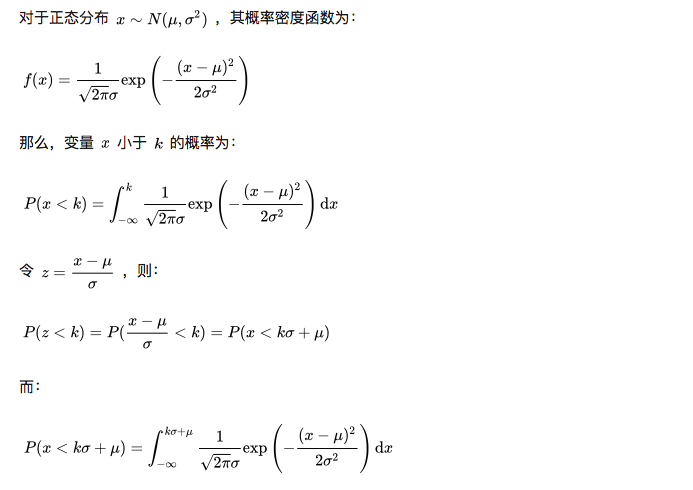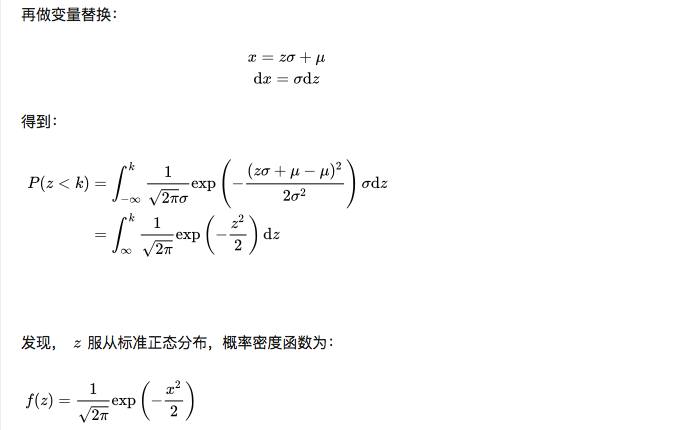# 普通正态分布如何转换到标准正态分布

## 1.普通正态分布转换标准正态分布公式

$z = \frac{X-\mu}{\sigma}$

## 2.证明## 3.几个应用的例子

3.1 假设公共汽车门的高度按成年男性碰头机会小于1%来设计。又假设成年男性的身高服从正态分布$X \sim N(170, 6^2)$，求问车门的高度$h$为多少?

$P(x > h) = 0.01$

$1 - P(x \le h) = 0.01$
$P(x \le h) = 0.99$

3.2 现在有一个$\mu = 10$$\sigma = 2$的正态随机变量，求x在10与14之间的概率是多少？

$P(0 \le z \le 2) = P(z \le 2) - P(z \le 0) = 0.4772$